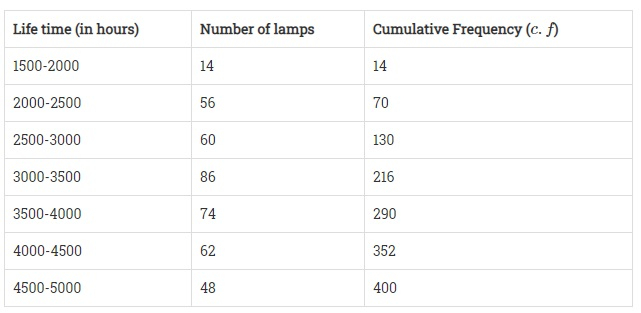Life time: (in hours)Number of lamps1500-2000142000-2500562500-3000603000-3500863500-4000744000-4500624500-500048
Find the median lifetime of a lamp."">

# The following table gives the distribution of the lifetime of 400 neon lamps:Life time: (in hours)Number of lamps1500-2000142000-2500562500-3000603000-3500863500-4000744000-4500624500-500048Find the median lifetime of a lamp."

Given:

The given table gives the distribution of the lifetime of 400 neon lamps.

To do:

We have to find the median life.

Solution:Here,

$N = 400$

$\frac{N}{2} = \frac{400}{2} = 200$

The cumulative frequency just greater than $\frac{N}{2}$ is 216 and the corresponding class is 3000 – 3500.

This implies, that 3000– 3500 is the median class.

Therefore,

$l = 3000, f = 86, F = 130$ and $h = (3500 - 3000) = 500$

Median $=\mathrm{l}+\frac{\frac{\mathrm{N}}{2}-\mathrm{F}}{\mathrm{f}} \times \mathrm{h}$

$=3000+\frac{200-130}{86} \times 500$

$=3000+\frac{70}{86} \times 500$

$=3000+\frac{35000}{86}$

$= 3000 + 406.98$

$= 3406.98$

The median life is 3406.98 hours.

Updated on: 10-Oct-2022

35 Views